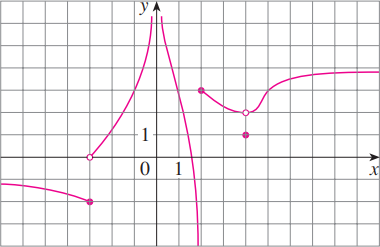×

×

# The graph of f is given. (a) Find each limit, or explain why it does not exist. (i) limISBN: 9781305270336 484

## Solution for problem 1 Chapter 2

Single Variable Calculus: Early Transcendentals | 8th Edition

• Textbook Solutions
• 2901 Step-by-step solutions solved by professors and subject experts
• Get 24/7 help from StudySoup virtual teaching assistantsSingle Variable Calculus: Early Transcendentals | 8th Edition

4 5 1 340 Reviews
20
3
Problem 1

The graph of f is given. (a) Find each limit, or explain why it does not exist. (i) lim xl21 fsxd (ii) lim xl231 fsxd (iii) lim xl23 fsxd (iv) lim xl4 fsxd (v) lim xl0 fsxd (vi) lim xl22 fsxd (vii) lim xl fsxd (viii) lim xl2 fsxd (b) State the equations of the horizontal asymptotes. (c) State the equations of the vertical asymptotes. (d) At what numbers is f discontinuous? Explain.

Step-by-Step Solution:

Problem 1

The graph of f is given(a) Find each limit or explain why it does not exist.

(i)(ii)(iii)(iv)(v)(vi)(vii)(viii)(b) State the equations of the horizontal asymptote.

(c) State the equations of the vertical asymptotes.

(d) At what numbers is f discontinuous? Explain.

Step by Step Solution

Step 1 of 6

(a)

(i)

The graph shows that the limitStep 2 of 6

Step 3 of 6

##### ISBN: 9781305270336

Unlock Textbook Solution